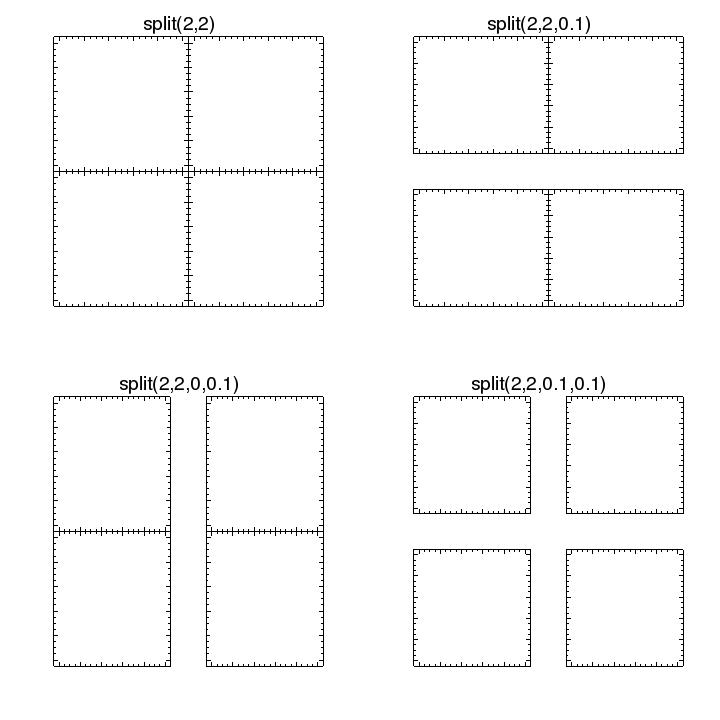## Can I have a grid of m by n plots?

The strip_chart command, described in the Multiple plots with a common axis entry, creates a single column or row of plots with a common axis. The split command is used to create an n by m grid of plots.

chips> split(3)


creates one column of three plots with no gaps between the plots.

chips> split(2, 3)


creates two columns of three rows, again with no gaps between the plots.

chips> split(2, 2, 0.1)


creates a two by two arrangement but this time adds a vertical gap between the two rows (but no gap between the two columns).

chips> split(2, 2, 0, 0.1)


is similar but has a gap between the columns rather than rows.

chips> split(2, 2, 0.1, 0.1)


creates the grid of plots with both horizontal and vertical spaces.

The arrangement of a two-by-two grid is shown in the following image:### Differences with strip_chart()

The main differences to the strip_chart command are:

• The split command can be used to make a n by m arrangement whereas strip_chart creates either a single row or column of plots.

• The plots created by split do not have any axes, whereas those from strip_chart. Also, the common axis of the plots in the latter case are "bound" together so will always display the same data range and use the same scaling.

• split will only create plots if necesary, re-using any existing plots, which means you can use it to add plots to a visualization, or to rearrange the existing plots. The strip_chart command always creates a new frame and uses this to create the new plots.